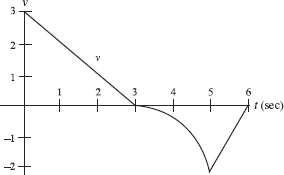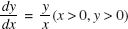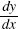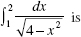# AP Calculus BC Practice Test 28

### Test Information9 questions18 minutes

Calculator Disallowed

1. Use the graph below, consisting of two line segments and a quarter-circle. The graph shows the velocity of an object during a 6-second interval.For how many values of t in the interval 0 < t < 6 is the acceleration undefined?

2. Use the graph below, consisting of two line segments and a quarter-circle. The graph shows the velocity of an object during a 6-second interval.During what time interval (in sec) is the speed increasing?

3. Ifand y = 3 when x = 1, then

4. A solid is cut out of a sphere of radius 2 by two parallel planes each 1 unit fromthe center. The volume of this solid is

5. Which one of the following improper integrals converges?

6. Let f (x) = x5 + 3x - 2, and let f -1 denote the inverse of f. Then (f -1) (2) equals

7. Find the domain of the particular solution of= 1 + y2 that passes through the origin.

8. Which of the following statements is (are) true about the graph of y = ln (4 + x2)?

I. It is symmetric to the y-axis.

II. It has a local minimum at x = 0.

III. It has inflection points at x = ±2.

9.